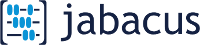version 0.0.3
April 1, 2020version 0.0.3
April 1, 2020

Location
Province:
Ss:
Sr:
Density
Snow density, γ:
kN/m3

#### Factors

Importance factor
Is:
Factors
Cb:
Cw:Length, b:
m
Height, h:
m

#### Roof geometry

Pitch:
/12
Slippery:
Thinking...

[4.1.6.2]
S = Is[Ss(CbCwCsCa)+Sr]

#### Factors

User input values:
Ss = 1
Sr = 1
Importance Factors
ULS: Is = 1.25
SLS: Is = 0.9
Roof slope = 0 degrees
Slope Factor
For non-slippery roof:
Slope <= 30 degrees.
Cs = 1
Cb = 0.8
Density = γ = 3 kN/m3
[Structural
Commentaries
Fig G-8]

### Drift Factors & Distribution

Ca(0) = 0.67*γ h/(CbSs = 2.513
a) when Ca(0) < ( 0.8/Cb=1 ) : Ca(0)=0.8/Cb
b) when Ca(0) > ( 2/Cb=2.5 ): Ca(0)=2/Cb

#### Ca(0) = 2.5

xd = 2h = 2 m. But 3 m ≤ xd ≤ 9 m:

#### xd = 3 m

h' = h - CbCwSs/γ = 0.733m

Min dist where (Cw = 1.0) = 10*h' = 7.333 m

Obstruction effect limit = 3 Ss / γ = 1 m

( b = 10 m ) > ( 3*Ss / γ = 1 m )

Cw = 1
Ca(0) = 2.5

#### 0 < x ≤ ( xd = 3m )

Cw = 1
Ca(x) decreases linearly over 0 < x ≤ xd
Ca(x) = 2.5 - 0.5 * x   (with x in meters)

Cw = 1
Ca(xd) = 1

Cw = 1.0
Ca(x) = 1

#### SULS = 1.25[1(0.8*1.0*1*1)+1] = 2.25 kPa

##### SSLS = 0.9[1(0.8*1.0*1*1)+1] = 1.62 kPa

By using Jabacus or information derived from this Service, you have agreed to the Terms of Service.

This information must be read or used in conjunction with Part 4 and the Structural Commentaries of the NBCC 2005. In the event of discrepancies, Part 4 and the Structural Commentaries of the NBCC 2005 shall govern.